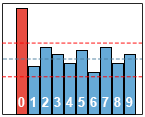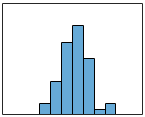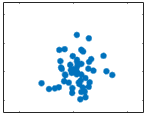Scatter-plotterThe pure mathematical proof.

You can open the instrument in a new window/tab.

Last digit short moduleThe Last Digit

In a random set of integer values, the last digit should occur with equal probability: 1/10. Deviation from randomness might be legit or might be a sign of artificial interference.

Method Description

Last Digit Analyzer in the Lab

Histo short moduleHistogram

The values under study are aggregated by intervals (bins). The aggregated sums are proportional to the heights of the bars. Most of the expected distributions are expected to be unimodal.

Method Description

Histogram Generator in Laboratory

Scatter-plot short moduleScatter-plot

Scatter-plot diagram visualizes correlations of two chosen values. Each pair of values, most often they are a subset of voting results by PECs, corresponds to a point whose coordinates are equal to the values. Uniform distributions are expected, without clusters, lines, abnormalities and artefacts.

Method Description

Scatter-Plotter in the Lab